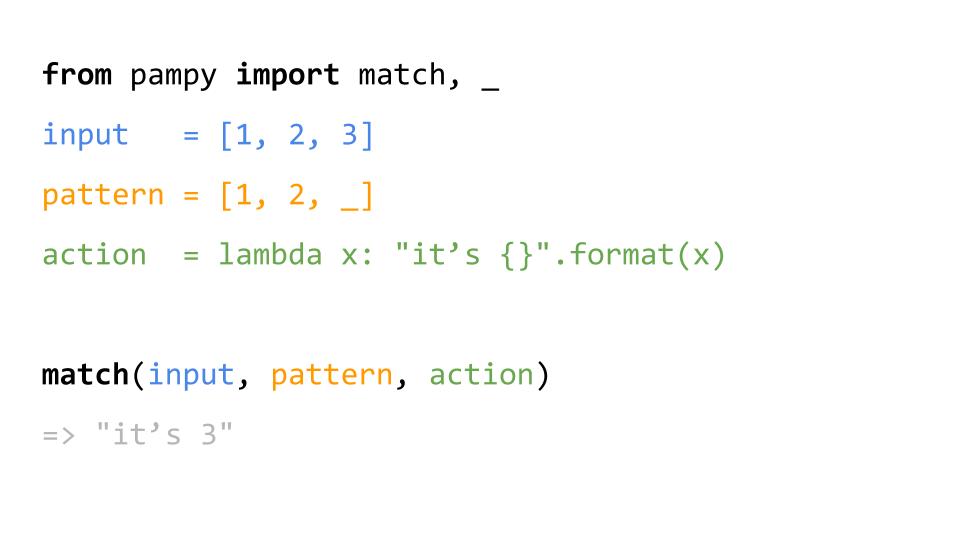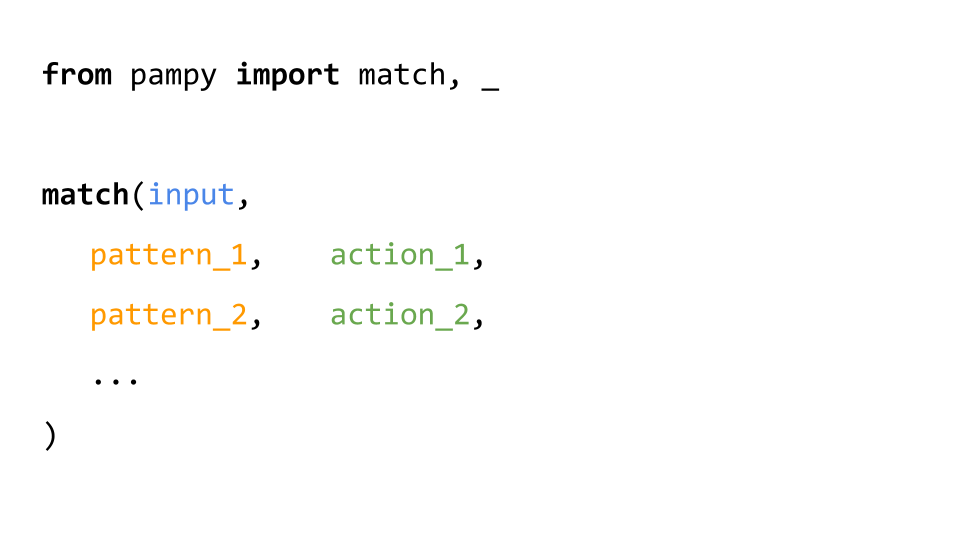# santinic / pampy

Pampy: The Pattern Matching for Python you always dreamed of.
Python
Latest commit 665c6b8 Jul 13, 2019
Type Name Latest commit message Commit time
Failed to load latest commit information.imgs Nov 9, 2018pampy Jul 13, 2019tests Mar 10, 2019.coveralls.yml Nov 9, 2018.gitignore Nov 9, 2018.travis.yml Dec 24, 2018LICENSE Nov 8, 2018README.md Mar 10, 2019setup.py Nov 9, 2018# Pampy: Pattern Matching for Python

Pampy is pretty small (150 lines), reasonably fast, and often makes your code more readable and hence easier to reason about. There is also a JavaScript version, called Pampy.js.## You can write many patterns

Patterns are evaluated in the order they appear.## You can write Fibonacci

The operator _ means "any other case I didn't think of".

```from pampy import match, _

def fibonacci(n):
return match(n,
1, 1,
2, 1,
_, lambda x: fibonacci(x-1) + fibonacci(x-2)
)```

## You can write a Lisp calculator in 5 lines

```from pampy import match, REST, _

def lisp(exp):
return match(exp,
int,                lambda x: x,
callable,           lambda x: x,
(callable, REST),   lambda f, rest: f(*map(lisp, rest)),
tuple,              lambda t: list(map(lisp, t)),
)

plus = lambda a, b: a + b
minus = lambda a, b: a - b
from functools import reduce

lisp((plus, 1, 2))                 	# => 3
lisp((plus, 1, (minus, 4, 2)))     	# => 3
lisp((reduce, plus, (range, 10)))       # => 45```

## You can match so many things!

```match(x,
3,              "this matches the number 3",

int,            "matches any integer",

(str, int),     lambda a, b: "a tuple (a, b) you can use in a function",

[1, 2, _],      "any list of 3 elements that begins with [1, 2]",

{'x': _},       "any dict with a key 'x' and any value associated",

_,              "anything else"
)```

## You can match [HEAD, TAIL]

```from pampy import match, HEAD, TAIL, _

x = [1, 2, 3]

match(x, [1, TAIL],     lambda t: t)            # => [2, 3]

match(x, [HEAD, TAIL],  lambda h, t: (h, t))    # => (1, [2, 3])
```

`TAIL` and `REST` actually mean the same thing.

## You can nest lists and tuples

```from pampy import match, _

x = [1, [2, 3], 4]

match(x, [1, [_, 3], _], lambda a, b: [1, [a, 3], b])           # => [1, [2, 3], 4]```

## You can nest dicts. And you can use _ as key!

```pet = { 'type': 'dog', 'details': { 'age': 3 } }

match(pet, { 'details': { 'age': _ } }, lambda age: age)        # => 3

match(pet, { _ : { 'age': _ } },        lambda a, b: (a, b))    # => ('details', 3)```

It feels like putting multiple _ inside dicts shouldn't work. Isn't ordering in dicts not guaranteed ? But it does because in Python 3.7, dict maintains insertion key order by default

## You can match class hierarchies

```class Pet:          pass
class Dog(Pet):     pass
class Cat(Pet):     pass
class Hamster(Pet): pass

def what_is(x):
return match(x,
Dog, 		'dog',
Cat, 		'cat',
Pet, 		'any other pet',
_, 		'this is not a pet at all',
)

what_is(Cat())      # => 'cat'
what_is(Dog())      # => 'dog'
what_is(Hamster())  # => 'any other pet'
what_is(Pet())      # => 'any other pet'
what_is(42)         # => 'this is not a pet at all'```

## Using Dataclasses

Pampy supports Python 3.7 dataclasses. You can pass the operator `_` as arguments and it will match those fields.

```@dataclass
class Pet:
name: str
age: int

pet = Pet('rover', 7)

match(pet, Pet('rover', _), lambda age: age)                    # => 7
match(pet, Pet(_, 7), lambda name: name)                        # => 'rover'
match(pet, Pet(_, _), lambda name, age: (name, age))            # => ('rover', 7)```

## Using typing

Pampy supports typing annotations.

```class Pet:          pass
class Dog(Pet):     pass
class Cat(Pet):     pass
class Hamster(Pet): pass

timestamp = NewType("year", Union[int, float])

def annotated(a: Tuple[int, float], b: str, c: E) -> timestamp:
pass

match((1, 2), Tuple[int, int], lambda a, b: (a, b))             # => (1, 2)
match(1, Union[str, int], lambda x: x)                          # => 1
match('a', Union[str, int], lambda x: x)                        # => 'a'
match('a', Optional[str], lambda x: x)                          # => 'a'
match(None, Optional[str], lambda x: x)                         # => None
match(Pet, Type[Pet], lambda x: x)                              # => Pet
match(Cat, Type[Pet], lambda x: x)                              # => Cat
match(Dog, Any, lambda x: x)                                    # => Dog
match(Dog, Type[Any], lambda x: x)                              # => Dog
match(15, timestamp, lambda x: x)                               # => 15
match(10.0, timestamp, lambda x: x)                             # => 10.0
match([1, 2, 3], List[int], lambda x: x)                        # => [1, 2, 3]
match({'a': 1, 'b': 2}, Dict[str, int], lambda x: x)            # => {'a': 1, 'b': 2}
match(annotated,
Callable[[Tuple[int, float], str, Pet], timestamp], lambda x: x
)                                                               # => annotated```

For iterable generics actual type of value is guessed based on the first element.

```match([1, 2, 3], List[int], lambda x: x)                        # => [1, 2, 3]
match([1, "b", "a"], List[int], lambda x: x)                    # => [1, "b", "a"]
match(["a", "b", "c"], List[int], lambda x: x)                  # raises MatchError
match(["a", "b", "c"], List[Union[str, int]], lambda x: x)      # ["a", "b", "c"]

match({"a": 1, "b": 2}, Dict[str, int], lambda x: x)            # {"a": 1, "b": 2}
match({"a": 1, "b": "dog"}, Dict[str, int], lambda x: x)        # {"a": 1, "b": "dog"}
match({"a": 1, 1: 2}, Dict[str, int], lambda x: x)              # {"a": 1, 1: 2}
match({2: 1, 1: 2}, Dict[str, int], lambda x: x)                # raises MatchError
match({2: 1, 1: 2}, Dict[Union[str, int], int], lambda x: x)    # {2: 1, 1: 2}```

Iterable generics also match with any of their subtypes.

```match([1, 2, 3], Iterable[int], lambda x: x)                     # => [1, 2, 3]
match({1, 2, 3}, Iterable[int], lambda x: x)                     # => {1, 2, 3}
match(range(10), Iterable[int], lambda x: x)                     # => range(10)

match([1, 2, 3], List[int], lambda x: x)                         # => [1, 2, 3]
match({1, 2, 3}, List[int], lambda x: x)                         # => raises MatchError
match(range(10), List[int], lambda x: x)                         # => raises MatchError

match([1, 2, 3], Set[int], lambda x: x)                          # => raises MatchError
match({1, 2, 3}, Set[int], lambda x: x)                          # => {1, 2, 3}
match(range(10), Set[int], lambda x: x)                          # => raises MatchError```

For Callable any arg without annotation treated as Any.

```def annotated(a: int, b: int) -> float:
pass

def not_annotated(a, b):
pass

def partially_annotated(a, b: float):
pass

match(annotated, Callable[[int, int], float], lambda x: x)     # => annotated
match(not_annotated, Callable[[int, int], float], lambda x: x) # => raises MatchError
match(not_annotated, Callable[[Any, Any], Any], lambda x: x)   # => not_annotated
match(annotated, Callable[[Any, Any], Any], lambda x: x)       # => raises MatchError
match(partially_annotated,
Callable[[Any, float], Any], lambda x: x
)                                                              # => partially_annotated```

TypeVar is not supported.

## All the things you can match

As Pattern you can use any Python type, any class, or any Python value.

The operator `_` and built-in types like `int` or `str`, extract variables that are passed to functions.

Types and Classes are matched via `instanceof(value, pattern)`.

`Iterable` Patterns match recursively through all their elements. The same goes for dictionaries.

Pattern Example What it means Matched Example Arguments Passed to function NOT Matched Example
`"hello"` only the string `"hello"` matches `"hello"` nothing any other value
`None` only `None` `None` nothing any other value
`int` Any integer `42` `42` any other value
`float` Any float number `2.35` `2.35` any other value
`str` Any string `"hello"` `"hello"` any other value
`tuple` Any tuple `(1, 2)` `(1, 2)` any other value
`list` Any list `[1, 2]` `[1, 2]` any other value
`MyClass` Any instance of MyClass. And any object that extends MyClass. `MyClass()` that instance any other object
`_` Any object (even None) that value
`ANY` The same as `_` that value
`(int, int)` A tuple made of any two integers `(1, 2)` `1` and `2` (True, False)
`[1, 2, _]` A list that starts with 1, 2 and ends with any value `[1, 2, 3]` `3` `[1, 2, 3, 4]`
`[1, 2, TAIL]` A list that start with 1, 2 and ends with any sequence `[1, 2, 3, 4]` `[3, 4]` `[1, 7, 7, 7]`
`{'type':'dog', age: _ }` Any dict with `type: "dog"` and with an age `{"type":"dog", "age": 3}` `3` `{"type":"cat", "age":2}`
`{'type':'dog', age: int }` Any dict with `type: "dog"` and with an `int` age `{"type":"dog", "age": 3}` `3` `{"type":"dog", "age":2.3}`
`re.compile('(\w+)-(\w+)-cat\$')` Any string that matches that regular expression expr `"my-fuffy-cat"` `"my"` and `"puffy"` `"fuffy-dog"`
`Pet(name=_, age=7)` Any Pet dataclass with `age == 7` `Pet('rover', 7)` `['rover']` `Pet('rover', 8)`
`Any` The same as `_` that value
`Union[int, float, None]` Any integer or float number or None `2.35` `2.35` any other value
`Optional[int]` The same as `Union[int, None]` `2` `2` any other value
`Type[MyClass]` Any subclass of MyClass. And any class that extends MyClass. `MyClass` that class any other object
`Callable[[int], float]` Any callable with exactly that signature `def a(q:int) -> float: ...` that function `def a(q) -> float: ...`
`Tuple[MyClass, int, float]` The same as `(MyClass, int, float)`
`Mapping[str, int]` Any subtype of `Mapping` acceptable too any mapping or subtype of mapping with string keys and integer values `{'a': 2, 'b': 3}` that dict `{'a': 'b', 'b': 'c'}`
`Iterable[int]` Any subtype of `Iterable` acceptable too any iterable or subtype of iterable with integer values `range(10)` and `[1, 2, 3]` that iterable `['a', 'b', 'v']`

## Using default

By default `match()` is strict. If no pattern matches, it raises a `MatchError`.

You can instead provide a fallback value using `default` to be used when nothing matches.

``````>>> match([1, 2], [1, 2, 3], "whatever")
MatchError: '_' not provided. This case is not handled: [1, 2]

>>> match([1, 2], [1, 2, 3], "whatever", default=False)
False
``````

## Using Regular Expressions

Pampy supports Python's Regex. You can pass a compiled regex as pattern, and Pampy is going to run `patter.search()`, and then pass to the action function the result of `.groups()`.

```def what_is(pet):
return match(pet,
re.compile('(\w+)-(\w+)-cat\$'),     lambda name, my: 'cat '+name,
re.compile('(\w+)-(\w+)-dog\$'),     lambda name, my: 'dog '+name,
_,                                  "something else"
)

what_is('fuffy-my-dog')     # => 'dog fuffy'
what_is('puffy-her-dog')    # => 'dog puffy'
what_is('carla-your-cat')   # => 'cat carla'
what_is('roger-my-hamster') # => 'something else'```

## Install for Python3

Pampy works in Python >= 3.6 Because dict matching can work only in the latest Pythons.

To install it:

`\$ pip install pampy`

or `\$ pip3 install pampy`

## If you really must use Python2

Pampy is Python3-first, but you can use most of its features in Python2 via this backport by Manuel Barkhau:

`pip install backports.pampy`

`from backports.pampy import match, HEAD, TAIL, _`
You can’t perform that action at this time.